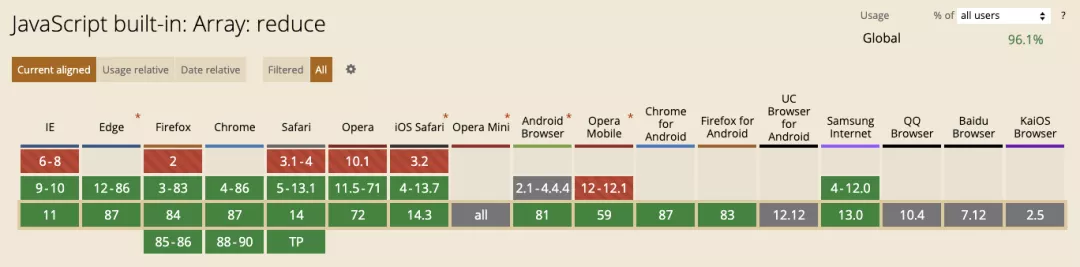Javascript的reduce函数用法

## 语法

`reduce` 接收 2 个参数：第一个参数是回调函数（必选），第二个参数是初始值 `initialValue`（可选） 。而第一个参数（回调函数），接收下面四个参数：

• Accumulator (acc) (累计器)
• Current Value (cur) (当前值)
• Current Index (idx) (当前索引)
• Source Array (src) (源数组)

#### 浏览器支持情况#### 不带初始值

``````[1,2,3,4].reduce((acc, cur) => {
return acc + cur
})
// 1 + 2 + 3 + 4
// 10
``````

#### 带初始值

``````[1,2,3,4].reduce((acc, cur) => {
return acc + cur
}, 10)
// 10 + 1 + 2 + 3 + 4
// 20
``````

⚠️ 初始值 `initialValue` 可以是任意类型。如果没有提供 `initialValue``reduce` 会从索引 1 的地方开始执行 `callback` 方法，跳过第一个索引。如果提供 `initialValue`，从索引 0 开始。它的执行就像一个贪吃蛇，蛇每吃一个豆子，豆子将会变成蛇身的一部分，蛇再去吃下一个豆子。

## 重塑

#### reduce -> map

`map` 方法接收一个回调函数，函数内接收三个参数，当前项、索引、原数组，返回一个新的数组，并且数组长度不变。知道了这些特征之后，我们用 `reduce` 重塑 `map`

``````const testArr = [1, 2, 3, 4]
Array.prototype.reduceMap = function(callback) {
return this.reduce((acc, cur, index, array) => {
const item = callback(cur, index, array)
acc.push(item)
return acc
}, [])
}
testArr.reduceMap((item, index) => {
return item + index
})
// [1, 3, 5, 7]
``````

`Array` 的原型链上添加 `reduceMap` 方法，接收一个回调函数 `callback` 作为参数（就是 `map` 传入的回调函数），内部通过 `this` 拿到当前需要操作的数组，这里 `reduce` 方法的第二个参数初始值很关键，需要设置成一个 `[]` ，这样便于后面把操作完的单项塞入 `acc` 。我们需要给 `callback` 方法传入三个值，当前项、索引、原数组，也就是原生 `map` 回调函数能拿到的值。返回 `item` 塞进 `acc`，并且返回 `acc` ，作为下一个循环的 `acc`（贪吃蛇原理）。最终 `this.reduce` 返回了新的数组，并且长度不变。

#### reduce -> forEach

`forEach` 接收一个回调函数作为参数，函数内接收四个参数当前项、索引、原函数、当执行回调函数 `callback` 时，用作 `this` 的值，并且不返回值。

``````const testArr = [1, 2, 3, 4]
Array.prototype.reduceForEach = function(callback) {
this.reduce((acc, cur, index, array) => {
callback(cur, index, array)
}, [])
}

testArr.reduceForEach((item, index, array) => {
console.log(item, index)
})
// 1234
// 0123
``````

#### reduce -> filter

`filter` 同样接收一个回调函数，回调函数返回 `true` 则返回当前项，反之则不返回。回调函数接收的参数同 `forEach`

``````const testArr = [1, 2, 3, 4]
Array.prototype.reduceFilter = function (callback) {
return this.reduce((acc, cur, index, array) => {
if (callback(cur, index, array)) {
acc.push(cur)
}
return acc
}, [])
}
testArr.reduceFilter(item => item % 2 == 0) // 过滤出偶数项。
// [2, 4]
``````

`filter` 方法中 `callback` 返回的是 `Boolean` 类型，所以通过 `if` 判断是否要塞入累计器 `acc` ，并且返回 `acc` 给下一次对比。最终返回整个过滤后的数组。

#### reduce -> find

`find` 方法中 `callback` 同样也是返回 `Boolean` 类型，返回你要找的第一个符合要求的项。

``````const testArr = [1, 2, 3, 4]
const testObj = [{ a: 1 }, { a: 2 }, { a: 3 }, { a: 4 }]
Array.prototype.reduceFind = function (callback) {
return this.reduce((acc, cur, index, array) => {
if (callback(cur, index, array)) {
if (acc instanceof Array && acc.length == 0) {
acc = cur
}
}
// 循环到最后若 acc 还是数组，且长度为 0，代表没有找到想要的项，则 acc = undefined
if ((index == array.length - 1) && acc instanceof Array && acc.length == 0) {
acc = undefined
}
return acc
}, [])
}
testArr.reduceFind(item => item % 2 == 0) // 2
testObj.reduceFind(item => item.a % 2 == 0) // {a: 2}
testObj.reduceFind(item => item.a % 9 == 0) // undefined
``````

## 衍生

#### 将二维数组转为一维数组

``````const testArr = [[1,2], [3,4], [5,6]]
testArr.reduce((acc, cur) => {
return acc.concat(cur)
}, [])
// [1,2,3,4,5,6]
``````

#### 计算数组中每个元素出现的个数

``````const testArr = [1, 3, 4, 1, 3, 2, 9, 8, 5, 3, 2, 0, 12, 10]
testArr.reduce((acc, cur) => {
if (!(cur in acc)) {
acc[cur] = 1
} else {
acc[cur] += 1
}
return acc
}, {})

// {0: 1, 1: 2, 2: 2, 3: 3, 4: 1, 5: 1, 8: 1, 9: 1, 10: 1, 12: 1}
``````

#### 按属性给数组分类

``````const bills = [
{ type: 'shop', momey: 223 },
{ type: 'study', momey: 341 },
{ type: 'shop', momey: 821 },
{ type: 'transfer', momey: 821 },
{ type: 'study', momey: 821 }
];
bills.reduce((acc, cur) => {
// 如果不存在这个键，则设置它赋值 [] 空数组
if (!acc[cur.type]) {
acc[cur.type] = [];
}
acc[cur.type].push(cur)
return acc
}, {})
``````

#### 数组去重

``````const testArr = [1,2,2,3,4,4,5,5,5,6,7]
testArr.reduce((acc, cur) => {
if (!(acc.includes(cur))) {
acc.push(cur)
}
return acc
}, [])
// [1, 2, 3, 4, 5, 6, 7]
``````

``````var arr = [
{key: 1, name: '张三'},
{key: 3, name: '李四'},
{key: 2, name: '王五'},
{key: 1, name: '赵六'}
];

let newobj = {};
arr = arr.reduce((preVal, curVal) => {
newobj[curVal.key] ? '' : newobj[curVal.key] = preVal.push(curVal);
return preVal
}, [])
``````

``````var uniqueVal = null;
arr = arr.filter(item => uniqueVal == item.key ? false : (uniqueVal = item.key) || true) // `或真`处理是修复key值为`非真`时，重复去重的BUG
``````

#### 求最大值或最小值

``````const testArr = [
{ age: 20 },
{ age: 21 },
{ age: 22 }
]
testArr.reduce((acc, cur) => {
if (!acc) {
acc = cur
return acc
}
if (acc.age < cur.age) {
acc = cur
return acc
}
return acc
}, 0)
// {age: 22}
``````

## 总结

`reduce` 的使用场景还有非常多，当你遇到数组复杂操作的时候，就是它大显身手的时候。深入研究它，对你今后的业务开发及面试都有很大的帮助。